# Mass-univariate twoway repeated measures ANOVA on single trial power¶

This script shows how to conduct a mass-univariate repeated measures ANOVA. As the model to be fitted assumes two fully crossed factors, we will study the interplay between perceptual modality (auditory VS visual) and the location of stimulus presentation (left VS right). Here we use single trials as replications (subjects) while iterating over time slices plus frequency bands for to fit our mass-univariate model. For the sake of simplicity we will confine this analysis to one single channel of which we know that it exposes a strong induced response. We will then visualize each effect by creating a corresponding mass-univariate effect image. We conclude with accounting for multiple comparisons by performing a permutation clustering test using the ANOVA as clustering function. The results final will be compared to multiple comparisons using False Discovery Rate correction.

```# Authors: Denis Engemann <denis.engemann@gmail.com>
#          Eric Larson <larson.eric.d@gmail.com>
#          Alexandre Gramfort <alexandre.gramfort@inria.fr>
#

import numpy as np
import matplotlib.pyplot as plt

import mne
from mne.time_frequency import tfr_morlet
from mne.stats import f_threshold_mway_rm, f_mway_rm, fdr_correction
from mne.datasets import sample

print(__doc__)
```

## Set parameters¶

```data_path = sample.data_path()
raw_fname = data_path + '/MEG/sample/sample_audvis_raw.fif'
event_fname = data_path + '/MEG/sample/sample_audvis_raw-eve.fif'
tmin, tmax = -0.2, 0.5

# Setup for reading the raw data

include = []

picks = mne.pick_types(raw.info, meg='grad', eeg=False, eog=True,

ch_name = 'MEG 1332'

event_id = dict(aud_l=1, aud_r=2, vis_l=3, vis_r=4)
epochs = mne.Epochs(raw, events, event_id, tmin, tmax,
reject=reject)
epochs.pick_channels([ch_name])  # restrict example to one channel
```

Out:

```Opening raw data file /home/circleci/mne_data/MNE-sample-data/MEG/sample/sample_audvis_raw.fif...
Read a total of 3 projection items:
PCA-v1 (1 x 102)  idle
PCA-v2 (1 x 102)  idle
PCA-v3 (1 x 102)  idle
Range : 25800 ... 192599 =     42.956 ...   320.670 secs
289 matching events found
Setting baseline interval to [-0.19979521315838786, 0.0] sec
Applying baseline correction (mode: mean)
3 projection items activated
Rejecting  epoch based on EOG : ['EOG 061']
Rejecting  epoch based on EOG : ['EOG 061']
Rejecting  epoch based on EOG : ['EOG 061']
Rejecting  epoch based on EOG : ['EOG 061']
Rejecting  epoch based on EOG : ['EOG 061']
Rejecting  epoch based on EOG : ['EOG 061']
Rejecting  epoch based on EOG : ['EOG 061']
Rejecting  epoch based on EOG : ['EOG 061']
Rejecting  epoch based on EOG : ['EOG 061']
Rejecting  epoch based on EOG : ['EOG 061']
Rejecting  epoch based on EOG : ['EOG 061']
Rejecting  epoch based on EOG : ['EOG 061']
Rejecting  epoch based on EOG : ['EOG 061']
Rejecting  epoch based on EOG : ['EOG 061']
Rejecting  epoch based on EOG : ['EOG 061']
Rejecting  epoch based on EOG : ['EOG 061']
Rejecting  epoch based on EOG : ['EOG 061']
Rejecting  epoch based on EOG : ['EOG 061']
Rejecting  epoch based on EOG : ['EOG 061']
Rejecting  epoch based on EOG : ['EOG 061']
Rejecting  epoch based on EOG : ['EOG 061']
Rejecting  epoch based on EOG : ['EOG 061']
Rejecting  epoch based on EOG : ['EOG 061']
Rejecting  epoch based on EOG : ['EOG 061']
Rejecting  epoch based on EOG : ['EOG 061']
Rejecting  epoch based on EOG : ['EOG 061']
Rejecting  epoch based on EOG : ['EOG 061']
Rejecting  epoch based on EOG : ['EOG 061']
Rejecting  epoch based on EOG : ['EOG 061']
Rejecting  epoch based on EOG : ['EOG 061']
Rejecting  epoch based on EOG : ['EOG 061']
Rejecting  epoch based on EOG : ['EOG 061']
Rejecting  epoch based on EOG : ['EOG 061']
Rejecting  epoch based on EOG : ['EOG 061']
Rejecting  epoch based on EOG : ['EOG 061']
Rejecting  epoch based on EOG : ['EOG 061']
Rejecting  epoch based on EOG : ['EOG 061']
Rejecting  epoch based on EOG : ['EOG 061']
Rejecting  epoch based on EOG : ['EOG 061']
Rejecting  epoch based on EOG : ['EOG 061']
Rejecting  epoch based on EOG : ['EOG 061']
Rejecting  epoch based on EOG : ['EOG 061']
Rejecting  epoch based on EOG : ['EOG 061']
Rejecting  epoch based on EOG : ['EOG 061']
Rejecting  epoch based on EOG : ['EOG 061']
Rejecting  epoch based on EOG : ['EOG 061']
Rejecting  epoch based on EOG : ['EOG 061']
Rejecting  epoch based on EOG : ['EOG 061']
Rejecting  epoch based on EOG : ['EOG 061']
Rejecting  epoch based on EOG : ['EOG 061']
Rejecting  epoch based on EOG : ['EOG 061']
Rejecting  epoch based on EOG : ['EOG 061']
Rejecting  epoch based on EOG : ['EOG 061']
Removing projector <Projection | PCA-v1, active : True, n_channels : 102>
Removing projector <Projection | PCA-v2, active : True, n_channels : 102>
Removing projector <Projection | PCA-v3, active : True, n_channels : 102>
```
Number of events 236 aud_l: 56aud_r: 60vis_l: 64vis_r: 56 -0.200 – 0.499 sec -0.200 – 0.000 sec

We have to make sure all conditions have the same counts, as the ANOVA expects a fully balanced data matrix and does not forgive imbalances that generously (risk of type-I error).

```epochs.equalize_event_counts(event_id)

# Factor to down-sample the temporal dimension of the TFR computed by
# tfr_morlet.
decim = 2
freqs = np.arange(7, 30, 3)  # define frequencies of interest
n_cycles = freqs / freqs
zero_mean = False  # don't correct morlet wavelet to be of mean zero
# To have a true wavelet zero_mean should be True but here for illustration
# purposes it helps to spot the evoked response.
```

Out:

```Dropped 12 epochs: 50, 92, 128, 147, 152, 154, 155, 186, 196, 198, 206, 207
```

## Create TFR representations for all conditions¶

```epochs_power = list()
for condition in [epochs[k] for k in event_id]:
this_tfr = tfr_morlet(condition, freqs, n_cycles=n_cycles,
decim=decim, average=False, zero_mean=zero_mean,
return_itc=False)
this_tfr.apply_baseline(mode='ratio', baseline=(None, 0))
this_power = this_tfr.data[:, 0, :, :]  # we only have one channel.
epochs_power.append(this_power)
```

Out:

```Not setting metadata
Applying baseline correction (mode: ratio)
Applying baseline correction (mode: ratio)
Applying baseline correction (mode: ratio)
Applying baseline correction (mode: ratio)
```

## Setup repeated measures ANOVA¶

We will tell the ANOVA how to interpret the data matrix in terms of factors. This is done via the factor levels argument which is a list of the number factor levels for each factor.

```n_conditions = len(epochs.event_id)
n_replications = epochs.events.shape // n_conditions

factor_levels = [2, 2]  # number of levels in each factor
effects = 'A*B'  # this is the default signature for computing all effects
# Other possible options are 'A' or 'B' for the corresponding main effects
# or 'A:B' for the interaction effect only (this notation is borrowed from the
# R formula language)
n_freqs = len(freqs)
times = 1e3 * epochs.times[::decim]
n_times = len(times)
```

Now we’ll assemble the data matrix and swap axes so the trial replications are the first dimension and the conditions are the second dimension.

```data = np.swapaxes(np.asarray(epochs_power), 1, 0)
# reshape last two dimensions in one mass-univariate observation-vector
data = data.reshape(n_replications, n_conditions, n_freqs * n_times)

# so we have replications * conditions * observations:
print(data.shape)
```

Out:

```(56, 4, 1688)
```

While the iteration scheme used above for assembling the data matrix makes sure the first two dimensions are organized as expected (with A = modality and B = location):

Sample data layout

trial

A1B1

A1B2

A2B1

B2B2

1

1.34

2.53

0.97

1.74

56

2.45

7.90

3.09

4.76

Now we’re ready to run our repeated measures ANOVA.

Note. As we treat trials as subjects, the test only accounts for time locked responses despite the ‘induced’ approach. For analysis for induced power at the group level averaged TRFs are required.

```fvals, pvals = f_mway_rm(data, factor_levels, effects=effects)

effect_labels = ['modality', 'location', 'modality by location']

# let's visualize our effects by computing f-images
for effect, sig, effect_label in zip(fvals, pvals, effect_labels):
plt.figure()
# show naive F-values in gray
plt.imshow(effect.reshape(8, 211), cmap=plt.cm.gray, extent=[times,
times[-1], freqs, freqs[-1]], aspect='auto',
origin='lower')
# create mask for significant Time-frequency locations
effect[sig >= 0.05] = np.nan
plt.imshow(effect.reshape(8, 211), cmap='RdBu_r', extent=[times,
times[-1], freqs, freqs[-1]], aspect='auto',
origin='lower')
plt.colorbar()
plt.xlabel('Time (ms)')
plt.ylabel('Frequency (Hz)')
plt.title(r"Time-locked response for '%s' (%s)" % (effect_label, ch_name))
plt.show()
```
•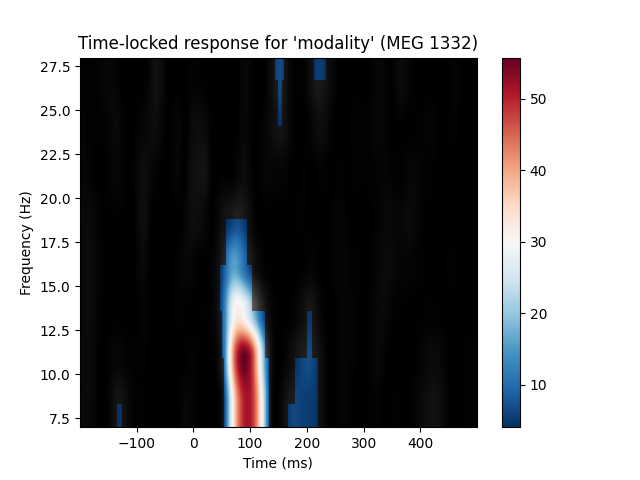•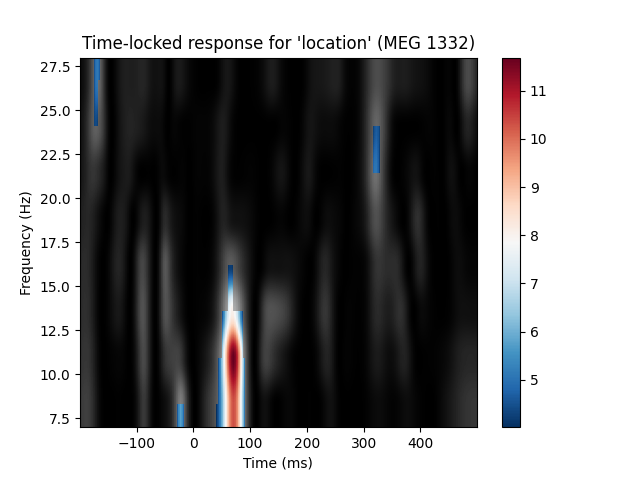•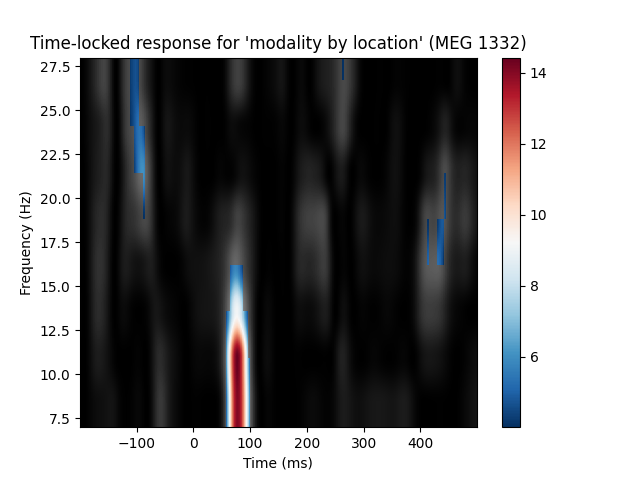## Account for multiple comparisons using FDR versus permutation clustering test¶

First we need to slightly modify the ANOVA function to be suitable for the clustering procedure. Also want to set some defaults. Let’s first override effects to confine the analysis to the interaction

```effects = 'A:B'
```

A stat_fun must deal with a variable number of input arguments. Inside the clustering function each condition will be passed as flattened array, necessitated by the clustering procedure. The ANOVA however expects an input array of dimensions: subjects X conditions X observations (optional). The following function catches the list input and swaps the first and the second dimension and finally calls the ANOVA function.

```def stat_fun(*args):
return f_mway_rm(np.swapaxes(args, 1, 0), factor_levels=factor_levels,
effects=effects, return_pvals=False)

# The ANOVA returns a tuple f-values and p-values, we will pick the former.
pthresh = 0.001  # set threshold rather high to save some time
f_thresh = f_threshold_mway_rm(n_replications, factor_levels, effects,
pthresh)
tail = 1  # f-test, so tail > 0
n_permutations = 256  # Save some time (the test won't be too sensitive ...)
T_obs, clusters, cluster_p_values, h0 = mne.stats.permutation_cluster_test(
epochs_power, stat_fun=stat_fun, threshold=f_thresh, tail=tail, n_jobs=1,
```

Out:

```stat_fun(H1): min=0.000001 max=14.419588
Running initial clustering
Found 1 clusters
Permuting 255 times...

0%|          |  : 0/255 [00:00<?,       ?it/s]
0%|          |  : 1/255 [00:00<00:08,   29.32it/s]
1%|1         |  : 3/255 [00:00<00:05,   44.53it/s]
2%|2         |  : 6/255 [00:00<00:04,   59.97it/s]
3%|3         |  : 8/255 [00:00<00:04,   59.76it/s]
4%|3         |  : 10/255 [00:00<00:04,   59.63it/s]
5%|4         |  : 12/255 [00:00<00:04,   59.53it/s]
5%|5         |  : 14/255 [00:00<00:04,   59.47it/s]
6%|6         |  : 16/255 [00:00<00:04,   59.42it/s]
7%|7         |  : 18/255 [00:00<00:03,   59.39it/s]
8%|7         |  : 20/255 [00:00<00:03,   59.35it/s]
9%|8         |  : 22/255 [00:00<00:03,   59.33it/s]
9%|9         |  : 23/255 [00:00<00:04,   56.10it/s]
9%|9         |  : 24/255 [00:00<00:04,   53.38it/s]
10%|#         |  : 26/255 [00:00<00:04,   53.95it/s]
11%|#         |  : 28/255 [00:00<00:04,   54.44it/s]
12%|#1        |  : 30/255 [00:00<00:04,   54.86it/s]
13%|#2        |  : 32/255 [00:00<00:04,   55.24it/s]
13%|#3        |  : 34/255 [00:00<00:03,   55.57it/s]
14%|#4        |  : 36/255 [00:00<00:03,   55.86it/s]
15%|#4        |  : 38/255 [00:00<00:03,   56.12it/s]
16%|#5        |  : 40/255 [00:00<00:03,   56.35it/s]
16%|#6        |  : 42/255 [00:00<00:03,   56.56it/s]
17%|#7        |  : 44/255 [00:00<00:03,   56.74it/s]
18%|#8        |  : 46/255 [00:00<00:03,   56.91it/s]
19%|#9        |  : 49/255 [00:00<00:03,   59.11it/s]
20%|##        |  : 51/255 [00:00<00:03,   59.12it/s]
21%|##        |  : 53/255 [00:00<00:03,   59.11it/s]
22%|##1       |  : 55/255 [00:00<00:03,   59.11it/s]
22%|##2       |  : 57/255 [00:00<00:03,   59.12it/s]
23%|##3       |  : 59/255 [00:01<00:03,   59.12it/s]
24%|##3       |  : 61/255 [00:01<00:03,   59.10it/s]
25%|##4       |  : 63/255 [00:01<00:03,   59.10it/s]
25%|##5       |  : 65/255 [00:01<00:03,   59.11it/s]
26%|##6       |  : 67/255 [00:01<00:03,   59.11it/s]
27%|##7       |  : 69/255 [00:01<00:03,   59.11it/s]
28%|##7       |  : 71/255 [00:01<00:03,   59.11it/s]
29%|##8       |  : 73/255 [00:01<00:03,   59.11it/s]
29%|##9       |  : 75/255 [00:01<00:03,   59.11it/s]
30%|###       |  : 77/255 [00:01<00:03,   59.10it/s]
31%|###       |  : 79/255 [00:01<00:02,   59.11it/s]
32%|###1      |  : 81/255 [00:01<00:02,   59.11it/s]
33%|###2      |  : 83/255 [00:01<00:02,   59.11it/s]
33%|###3      |  : 85/255 [00:01<00:02,   59.11it/s]
34%|###4      |  : 87/255 [00:01<00:02,   59.12it/s]
35%|###4      |  : 89/255 [00:01<00:02,   59.12it/s]
36%|###5      |  : 91/255 [00:01<00:02,   59.13it/s]
36%|###6      |  : 93/255 [00:01<00:02,   59.13it/s]
37%|###6      |  : 94/255 [00:01<00:02,   57.42it/s]
38%|###7      |  : 96/255 [00:01<00:02,   57.50it/s]
38%|###8      |  : 98/255 [00:01<00:02,   57.56it/s]
39%|###9      |  : 100/255 [00:01<00:02,   57.65it/s]
40%|###9      |  : 101/255 [00:01<00:02,   56.14it/s]
40%|####      |  : 103/255 [00:01<00:02,   56.30it/s]
41%|####1     |  : 105/255 [00:01<00:02,   56.45it/s]
42%|####1     |  : 107/255 [00:01<00:02,   56.60it/s]
43%|####2     |  : 109/255 [00:01<00:02,   56.73it/s]
44%|####3     |  : 111/255 [00:01<00:02,   56.86it/s]
44%|####4     |  : 113/255 [00:01<00:02,   56.98it/s]
45%|####5     |  : 116/255 [00:01<00:02,   58.64it/s]
46%|####6     |  : 118/255 [00:02<00:02,   58.66it/s]
47%|####7     |  : 120/255 [00:02<00:02,   58.68it/s]
48%|####7     |  : 122/255 [00:02<00:02,   58.71it/s]
49%|####8     |  : 124/255 [00:02<00:02,   58.72it/s]
49%|####9     |  : 126/255 [00:02<00:02,   58.74it/s]
50%|#####     |  : 128/255 [00:02<00:02,   58.76it/s]
51%|#####     |  : 130/255 [00:02<00:02,   58.77it/s]
52%|#####1    |  : 132/255 [00:02<00:02,   58.79it/s]
53%|#####2    |  : 134/255 [00:02<00:02,   58.81it/s]
53%|#####2    |  : 135/255 [00:02<00:02,   57.30it/s]
54%|#####3    |  : 137/255 [00:02<00:02,   57.39it/s]
54%|#####4    |  : 138/255 [00:02<00:02,   55.95it/s]
55%|#####4    |  : 140/255 [00:02<00:02,   56.10it/s]
55%|#####5    |  : 141/255 [00:02<00:02,   54.75it/s]
56%|#####6    |  : 143/255 [00:02<00:02,   54.96it/s]
56%|#####6    |  : 144/255 [00:02<00:02,   53.65it/s]
57%|#####7    |  : 146/255 [00:02<00:02,   53.92it/s]
58%|#####7    |  : 147/255 [00:02<00:02,   52.67it/s]
58%|#####8    |  : 149/255 [00:02<00:02,   52.99it/s]
59%|#####9    |  : 151/255 [00:02<00:01,   53.29it/s]
60%|######    |  : 153/255 [00:02<00:01,   53.58it/s]
61%|######    |  : 155/255 [00:02<00:01,   53.86it/s]
62%|######1   |  : 157/255 [00:02<00:01,   54.13it/s]
62%|######2   |  : 159/255 [00:02<00:01,   54.38it/s]
63%|######3   |  : 161/255 [00:02<00:01,   54.62it/s]
64%|######3   |  : 163/255 [00:02<00:01,   54.85it/s]
65%|######4   |  : 165/255 [00:02<00:01,   55.07it/s]
65%|######5   |  : 167/255 [00:02<00:01,   55.26it/s]
66%|######5   |  : 168/255 [00:02<00:01,   53.96it/s]
67%|######6   |  : 170/255 [00:03<00:01,   54.23it/s]
67%|######7   |  : 172/255 [00:03<00:01,   54.48it/s]
69%|######8   |  : 175/255 [00:03<00:01,   56.20it/s]
69%|######9   |  : 177/255 [00:03<00:01,   56.35it/s]
70%|#######   |  : 179/255 [00:03<00:01,   56.48it/s]
71%|#######   |  : 181/255 [00:03<00:01,   56.61it/s]
72%|#######1  |  : 183/255 [00:03<00:01,   56.74it/s]
73%|#######2  |  : 185/255 [00:03<00:01,   56.87it/s]
73%|#######3  |  : 187/255 [00:03<00:01,   56.98it/s]
74%|#######4  |  : 189/255 [00:03<00:01,   57.09it/s]
75%|#######4  |  : 191/255 [00:03<00:01,   57.19it/s]
76%|#######5  |  : 193/255 [00:03<00:01,   57.28it/s]
76%|#######6  |  : 195/255 [00:03<00:01,   57.37it/s]
77%|#######7  |  : 197/255 [00:03<00:01,   57.46it/s]
78%|#######8  |  : 199/255 [00:03<00:00,   57.54it/s]
79%|#######9  |  : 202/255 [00:03<00:00,   59.11it/s]
80%|########  |  : 204/255 [00:03<00:00,   59.11it/s]
81%|########  |  : 206/255 [00:03<00:00,   59.11it/s]
82%|########1 |  : 208/255 [00:03<00:00,   59.10it/s]
82%|########2 |  : 210/255 [00:03<00:00,   59.10it/s]
83%|########3 |  : 212/255 [00:03<00:00,   59.10it/s]
84%|########4 |  : 215/255 [00:03<00:00,   60.59it/s]
85%|########5 |  : 217/255 [00:03<00:00,   60.52it/s]
86%|########5 |  : 219/255 [00:03<00:00,   60.46it/s]
87%|########6 |  : 221/255 [00:03<00:00,   60.39it/s]
87%|########7 |  : 223/255 [00:03<00:00,   60.33it/s]
88%|########8 |  : 225/255 [00:03<00:00,   60.26it/s]
89%|########9 |  : 227/255 [00:03<00:00,   60.20it/s]
90%|########9 |  : 229/255 [00:03<00:00,   60.15it/s]
91%|######### |  : 231/255 [00:03<00:00,   60.10it/s]
91%|#########1|  : 233/255 [00:04<00:00,   60.05it/s]
93%|#########2|  : 236/255 [00:04<00:00,   61.49it/s]
93%|#########3|  : 238/255 [00:04<00:00,   61.36it/s]
94%|#########4|  : 240/255 [00:04<00:00,   61.26it/s]
95%|#########4|  : 242/255 [00:04<00:00,   61.15it/s]
96%|#########5|  : 244/255 [00:04<00:00,   61.06it/s]
96%|#########6|  : 246/255 [00:04<00:00,   60.96it/s]
97%|#########7|  : 248/255 [00:04<00:00,   60.87it/s]
98%|#########8|  : 250/255 [00:04<00:00,   60.78it/s]
99%|#########8|  : 252/255 [00:04<00:00,   60.70it/s]
100%|#########9|  : 254/255 [00:04<00:00,   60.62it/s]
100%|##########|  : 255/255 [00:04<00:00,   58.30it/s]
Computing cluster p-values
Done.
```

Create new stats image with only significant clusters:

```good_clusters = np.where(cluster_p_values < .05)
T_obs_plot = T_obs.copy()
T_obs_plot[~clusters[np.squeeze(good_clusters)]] = np.nan

plt.figure()
for f_image, cmap in zip([T_obs, T_obs_plot], [plt.cm.gray, 'RdBu_r']):
plt.imshow(f_image, cmap=cmap, extent=[times, times[-1],
freqs, freqs[-1]], aspect='auto',
origin='lower')
plt.xlabel('Time (ms)')
plt.ylabel('Frequency (Hz)')
plt.title("Time-locked response for 'modality by location' (%s)\n"
" cluster-level corrected (p <= 0.05)" % ch_name)
plt.show()
```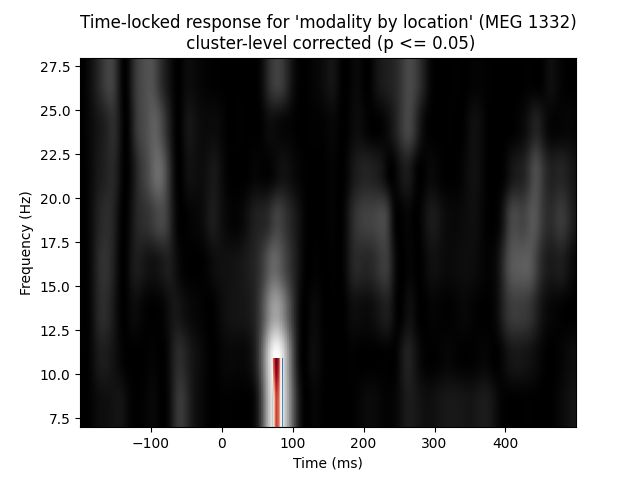Now using FDR:

```mask, _ = fdr_correction(pvals)
T_obs_plot2 = T_obs.copy()

plt.figure()
for f_image, cmap in zip([T_obs, T_obs_plot2], [plt.cm.gray, 'RdBu_r']):
if np.isnan(f_image).all():
continue  # nothing to show
plt.imshow(f_image, cmap=cmap, extent=[times, times[-1],
freqs, freqs[-1]], aspect='auto',
origin='lower')

plt.xlabel('Time (ms)')
plt.ylabel('Frequency (Hz)')
plt.title("Time-locked response for 'modality by location' (%s)\n"
" FDR corrected (p <= 0.05)" % ch_name)
plt.show()
```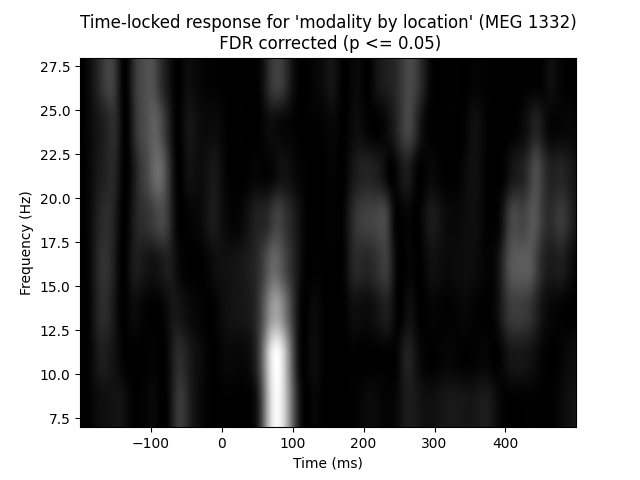Both cluster level and FDR correction help get rid of potential spots we saw in the naive f-images.

Total running time of the script: ( 0 minutes 21.423 seconds)

Estimated memory usage: 163 MB

Gallery generated by Sphinx-Gallery